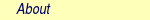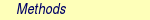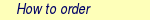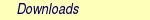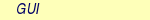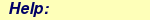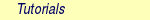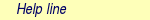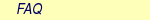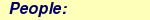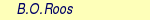MOLCAS manual:Next: 8.29 MCPDFT Up: 8. Programs Previous: 8.27 mckinley (a.k.a. denali)

Subsections

# 8.28 mclr

The mclr program in MOLCAS performs response calculations on single and multiconfigurational SCF wave functions with the technique described in . The right hand side (RHS) and thus the perturbation has to be defined through a preceding MCKINLEY calculation. Second order derivatives are obtained from a MCKINLEY and a consecutive mclr calculation, with a geometrical displacement as the external perturbation. If the response of a geometrical perturbation is calculated, harmonic frequencies corresponding to the most abundant masses are printed. MCLR also calculates isotope shifted frequencies. Per default, vibrational frequencies are calculated for all possible single isotopic substitutions. MCLR always calculates the response of an electric field and prints the polarizability.

Note that the user should not normally, for frequency calculations, request the execution of this module since this will be automatic.

The mclr code also calculates the Lagrangian multipliers required for a SA-MCSCF single state gradient , where the RHS is generated by the program itself. Through an mclr and a consecutive alaska calculation, analytical gradients of a SA-MCSCF state may be obtained. Note that alaska will automatically run the mclr module! Thus, with slapaf geometry optimizations of excited MCSCF states can be performed.

The MCLR program is based on the split GUGA formalism. However, it uses determinant based algorithms to solve the configuration interaction problem , in analogy to how it is done in the RASSCF. For spin symmetric wave function (MS=0) the time reversal symmetry is used, and the innermost loops are performed in combinations instead of determinant.

The upper limit to the size of the CI wave function that can be handled with the present program is about the same as for the RASSCF. The present version of the code is just able to handle CASSCF wave function, RASSCF wave function will soon be included.

The orbital handling is based on a one index transformation technique. The integrals is the transformed to occupied orbitals in two indexes, this can be done directly or from disk based integrals generated by seward.

## 8.28.1 Dependencies

To start the MCLR module the one-electron integrals generated by SEWARD have to be available. Moreover, MCLR requires the wave function from a SCF or RASSCF calculation and apart from in an evaluation of SA-MCSCF gradients, it also requires the differentiated integrals from MCKINLEY.

## 8.28.2 Files

### 8.28.2.1 Input files

MCLR will use the following input files: ONEINT, ORDINT,RUNFILE, ABDATA, RYSRW, JOBIPH (for more information see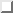).

### 8.28.2.2 Output files

 File Contents MCKINT Communication file between mclr and mckinley and rassi UNSYM ASCII file where all essential information, like geometry, Hessian normal modes and dipole derivatives are stored. MLDNFQ Molden input file for harmonic frequency analysis.

### 8.28.2.3 Scratch files

 File Contents TEMP0x x=1,8 used for for integral transformation and storing half transformed integrals. REORD Used for storing data used in the transformation of CI vectors from determinant base to CSF base. TEMPCIV Exchange file for temporary storing the CI vectors during the PCG. RESP Binary file where the solution of the response equations are stored. JOPR Used for half transformed integrals in direct mode. KOPR Used for half transformed integrals in direct mode.

## 8.28.3 Input

This section describes the input to the MCLR program in the MOLCAS program system. The input for each module is preceded by its name like:

&MCLR

### 8.28.3.1 Optional keywords

A list of these keywords is given below:
 Keyword Meaning SALA Makes MCLR compute the Lagrangian multipliers for a state average MCSCF wave function. These multipliers are required by ALASKA to obtain analytical gradients for an excited state, when the excited state is determined by a SA optimization. SALA has to be followed by an integer on the next line, specifying the excited state for which the gradient is required. NAC Like SALA, but for computing nonadiabatic couplings. It must be followed by two integers on the next line, specifying the states between which the coupling is required. Note that, unlike SALA, the numbering here is absolute, regardless of which roots are included in the state average. EXPDimension Here follows the dimension of the explicit Hamiltonian used as preconditioner in the Preconditioned conjugate gradient algorithm. Default 100. ITERations Specify the maximum number of iterations in the PCG. Default 200. LOWMemory Lowers the amount of memory used, by paging out the CI vectors on disk. This will lower the performance, but the program will need less memory. PRINt Raise the print level, default 0. RASSi This keyword is used for transforming the CI vectors to split GUGA representation, and transforming the orbital rotations to AO basis, to make the response accessible for state interaction calculations. SEWArd Specify one particle operators, used as right hand side, form the ONEINT file constructed by SEWARD The keyword is followed by one row for each perturbation: LABEL symmetry Component EndSeward Marks the end of perturbation specifications read from SEWARD ONEINT file. THREshold Specify the convergence threshold for the PCG. Default is 1.0e-4. DISOTOPE Calculates frequencies modified for double isotopic substitution. THERmochemistry Request an user specified thermochemical analysis. The keyword must be followed by a line containing the Rotational Symmetry Number, a line containing the Pressure (in atm), and lines containing the Temperatures (in K) for which the thermochemistry will be calculated. The section is ended by the keyword "End of PT". TIME Calculates the time dependent response of an electric periodic perturbation. The frequency of the perturbation should be specified on the following line. Used to calculated time dependent polarizabilities and required in a RASSI calculation of two photon transition moments. MASS Used to generate single and double (in conjunction with DISO) isotope shifted frequencies, with the isotope masses specified by the user. This implementation can be useful for example in calculating intermolecular frequencies which are contaminated by the BSSE. By setting the corresponding masses to the very large numbers, ghost orbitals can be used in the frequency calculation. MASS needs the atomic label and the new mass in units of u (real), for each element of the molecule.

### 8.28.3.2 Input example

A default input for a harmonic frequency calculation.

&MCLR

An input for a harmonic frequency calculation with modified isotopic masses for hydrogen and oxygen.

&MCLR
MASS
H  =  2.0079
O  =  150000.998

Thermochemistry for an asymmetric top (Rotational Symmetry Number = 1), at 1.0 atm and 273.15, 298.15, 398.15 and 498.15 K.

&MCLR
THERmochemistry
1
1.0
273.15  ;  298.15  ;  398.15  ;  498.15
End  of  PT

The time dependent response is calculated for a perturbation of frequency 0.2 au.

&MCLR
TIME  =  0.2
The time dependent response is calculated for a perturbation of frequency 0.2 au.

The input:

&MCLR
SALA  =  2
computes the Lagrangian multipliers for state number 2 in the SA root. Note, that 2 refers to the SA root. Thus, if the ground state is not included in the SA, the numbering of roots in the CI root and SA root differ. With the following RASSCF input:

&RASSCF
CiRoot
2  3
2  3
1  1
RlxRoot  =  2
SALA 2 yields the gradient for CI root number 3. Geometry optimization of an excited SA-CASSCF state can be done normally using EMIL commands, and requires the use of the RLXR keyword in the RASSCF input to specify the selected root to be optimized. An explicit input to MCLR is not required but can be specified if default options are not appropriate.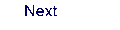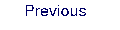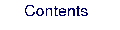Next: 8.29 MCPDFT Up: 8. Programs Previous: 8.27 mckinley (a.k.a. denali)